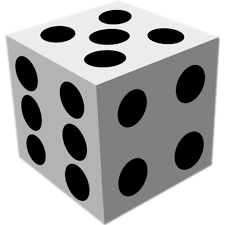# A six-sided

A six-sided die is rolled once. What is the probability that the number rolled is an even number greater than two?

p =  0.3333

### Step-by-step explanation:Did you find an error or inaccuracy? Feel free to write us. Thank you!

Tips for related online calculators
Need help calculating sum, simplifying, or multiplying fractions? Try our fraction calculator.
Would you like to compute the count of combinations?